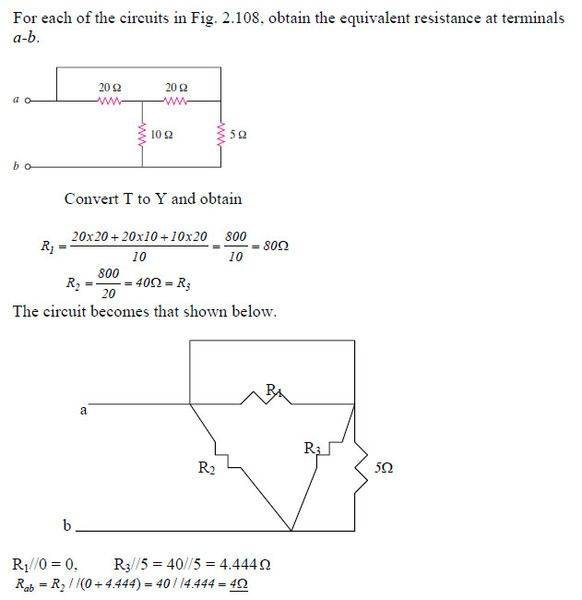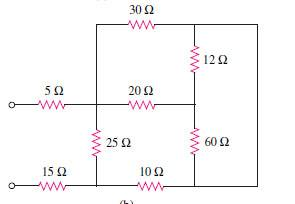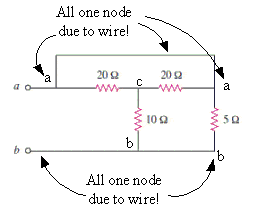# Current division, equivalent resistances and short circuts

Hi everyone, this my first post in here.

Anyway, this is a solved problem, but I can't get the answer :S

## Homework Statement## Homework Equations

* Series-Parallel combination (RT=R1+R2+R3...+Rn for series, 1/RT=1/R1+1/R2+1/R3...+1/Rn for parallel)

* WYE (TEE) - DELTA (PI) Transformations:

1- WYE (TEE) to DELTA (PI): Each resistor in the DELTA (PI) is equal to the sum of all possible products of WYE (TEE) resistors taken two at a time, divided by the opposite WYE (TEE) resistor.

2- DELTA (PI) to WYE (TEE): Each resistor in the WYE (TEE) is equal to the product of the resistors in two adjacent DELTA (PI) branches, divided by the sum of all three DELTA (PI) resistors.

## The Attempt at a Solution

I've tried to solve using two methods above, and I've ended up with 27.14 ohm for the equivalent resistance with both methods. When I looked at the solution above, I saw that the line the two 20 ohms resistors was not ignored (I though that as long as there is not resistor in a line, it can be ignored), but instead it was assumed that there was a 0 ohm resistor.

so my questions are:

1- How does the current flows or divides in the circuit? does the current flow normally through any line even if there isn't any resistor in it?

2- Is this case the same for the following?Thank you in advance.

## Answers and Replies

gneill
Mentor
A wire is the same as a zero ohm resistance. More importantly, any path created by continuous wiring comprises a single node. Connections of components to a node may be made anywhere, and you are free to move the connection points to anyplace along the continuous path. That means that you can reorganize the circuit diagram in any way you wish (to make more obvious series or parallel relationships) so long as the individual components retain their connections to the same nodes. Feel free to bend or stretch the wires to any shape you want, too.

Both of the circuits you show can be solved without resorting to Y or Δ transformations simply by reorganizing some of the components according to the above rules. Start by labeling the individual nodes so that you can recognize them and confirm that any component movements retain the same node affiliations.See if you can "move" the 5Ω resistor and the right-hand 20Ω resistor to new locations that will make obvious some series or parallel opportunities.

#### Attachments

I got the idea, thanks :)